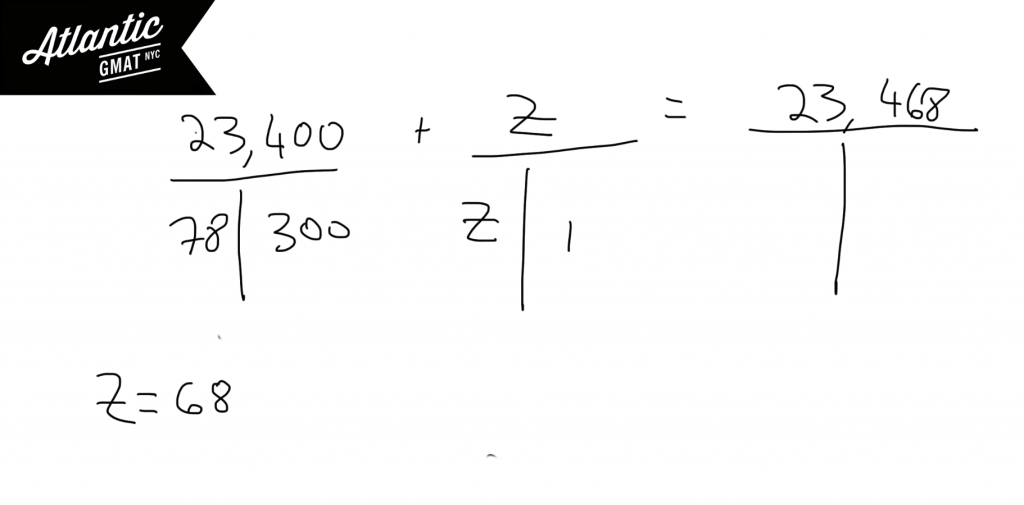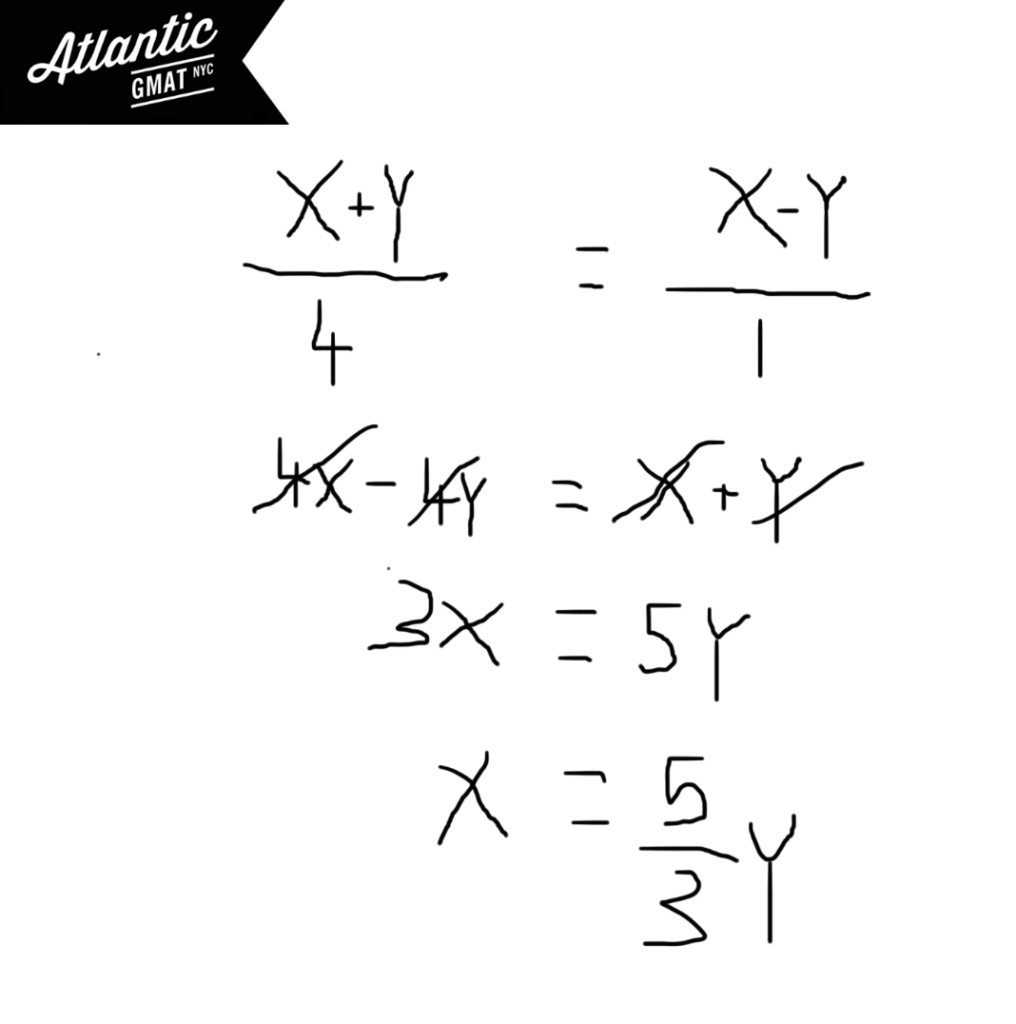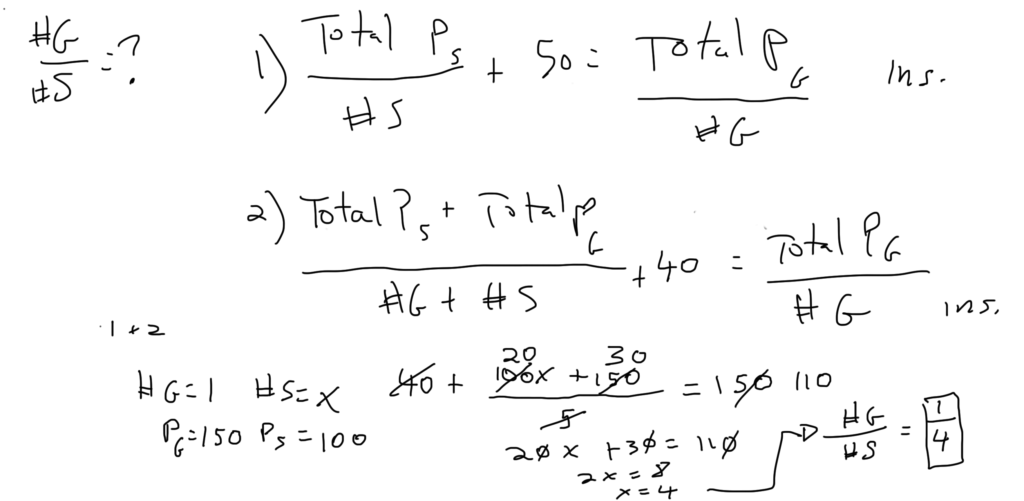Private GMAT Tutoring in NYC & Online

Last month 15 homes were sold in Town X. The average (arithmetic mean) sale price of the homes was \$150,000 and the median sale price was \$130,000. Which of the following statements must be true?

I. At least one of the homes was sold for more than \$165,000.
II. At least one of the homes was sold for more than \$130,0000 and less than \$150,000
III. At least one of the homes was sold for less than \$130,000.

(A) I only
(B) II only
(C) III only
(D) I and II
(E) I and III

GMAT Question of the Day - Problem Solving - Average/Word Problem

For the past 300 days machine X has produced an average of 78 units of part K. Today machine X produced Z units of part K bringing machine X's total production of part K to 23,468 units. What is the value of Z?

A. 34

B. 36

C. 68

D. 72

E. 74

GMAT Question of the Day Solution

GMAT weighted average questions are very similar to GMAT Rate/Work questions. For both question types you can use a T to organize the information. The T will help you make inferences. It can also help you to define the question. In a classic GMAT weighted average question you will have two scenarios and a total. You can organize those scenarios into three T's. Once you've done that this question of the day breaks down to simple arithmetic. Here's an Official GMAT question on which we also use the T method to organize the information: A boat traveled upstream a distance of 90 miles at an average speed of (v-3) miles per hour and then traveled the same distance downstream at an average speed of (v+3) miles per hourMore GMAT Average Rate Practice Questions:

Distance, Work and Rate - Average Rate 1

Distance, Work and Rate - Average Rate 2

For more GMAT distance, work, and rate practice visit GMAT Question of the Day.

GMAT Question of the Day - Problem Solving - Word Problem/Ratio/Algebra

Alice and Carl each completed a portion of a certain job. Alice completed 20% of the job while Carl completed the rest. If they were each paid the same amount, what proportion of her payment must Alice give to Carl so that they each are paid the amount of the total that is proportionate with the amount of work that they each performed?

A. 1/2

B. 2/3

C. 3/5

D. 3/4

E. 5/8

GMAT Question of the Day Solution

The GMAT Quant section has a whole bunch of word problems so it's important to feel comfortable with them. These questions tend to have a tough image but are often simple in concept (this question of the day has this feel). One thing that seems to help GMAT tutoring students: Taking time to carefully read the question. You do not need to solve the question as you read. You don't even have to take notes. The important thing is to process the information. To understand what the question is asking you to do. This is the time to make a broad plan for solving the question.

In this case Alice will need to give Carl part of her payment so that the pay per work is equal. Sounds like we can set up an equation with Carl on one side and Alice on the other. Pick a variable for the original payment (let's call it x) and pick a variable for the amount that Alice needs to lose from her side and that Carl will gain (let's call this y). Solve for y in terms of x and that will tell you the proportion of her payment that Alice needed to give to Carl.

You might be thinking: how do I deal with the different proportions of the job done by Alice and Carl? Well - look at it this way. We're dealing with 20% and 80% (.2 and .8). So the ratio is 1:4. Carl did 4 units of work to Alice's 1 unit of work. So Carl's money divided by his effort of 4 units must be equal to Alice's money divided by her effort of one unit.GMAT Question of the Day - Data Sufficiency - Ratio/Weighted Average

The ratio of the number of students in the math department, history department, and science department is 3 to 7 to 10 respectively. Is the average height of students from all of the departments less than 60 inches?

(1) The sum of the heights of all the students is less than 100 feet.

(2) This math department has 7 fewer students than the science department.

GMAT Question of the Day Solution

This GMAT question of the day presents another threshold question. In this case we want to know whether we are above or below 60 inches. From the given information we know that the minimum number of students is 20 (3 + 7 + 10).

Statement (1) If we divide max height (100 feet or 1,200 inches) by min students (20) we get 60. That's right at the threshold. Because we have an inequality we must be below this threshold. Sufficient.

Statement (2) From this we can infer the number of students but have no information on their heights. Insufficient.

GMAT Question of the Day - Data Sufficiency - Weighted Average/Ratios

Each Doctor at a certain medical practice is either a specialist or a generalist. What is the ratio of generalists to specialists?

1) The average (arithmetic mean) number of procedures performed per year by specialists is 50 less than the average (arithmetic mean) number of procedures performed per year by generalists.

2) The average (arithmetic mean) number of procedures performed by generalists is 40 more than the average (arithmetic mean) number of procedures performed by all of the doctors at the practice.

GMAT Question of the Day Solution:

The question is asking about ratios/proportions so think about picking numbers. It should be clear that each piece of information on it's own is insufficient. There are too many moving parts. Try a few different sets of numbers to prove this to yourself. Putting things together the results are locked into a ratio of 1:4. Again, pick numbers for this. You know that there must be more specialists than generalists so assume that there's only one generalist and that there are x specialists. Also from statement 1 you know that the average for generalists is 50 more than the average for specialists. So you can pick numbers to represent this. I chose 150 for the generalists and 100 for the specialists. Set up the equation and solve for x.CONTACT

Atlantic GMAT

405 East 51st St.

NY, NY 10022

(347) 669-3545

info@AtlanticGMAT.com